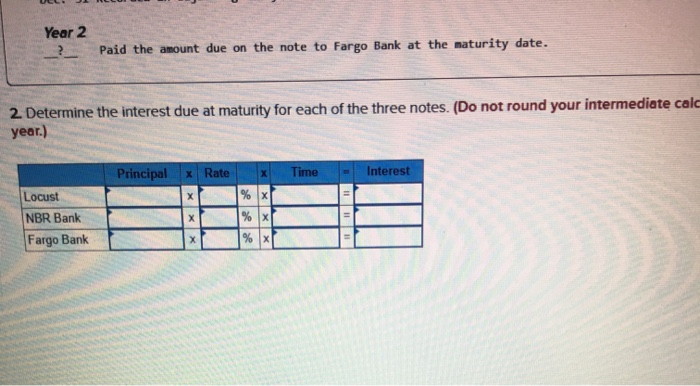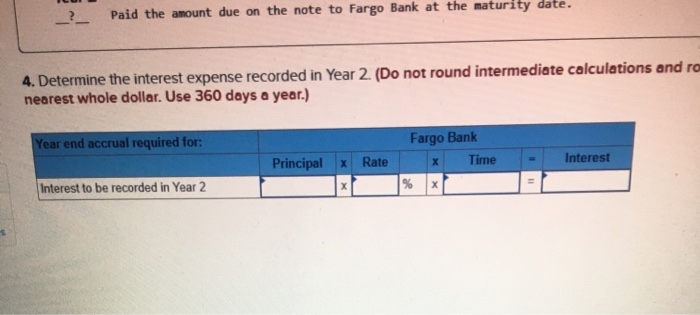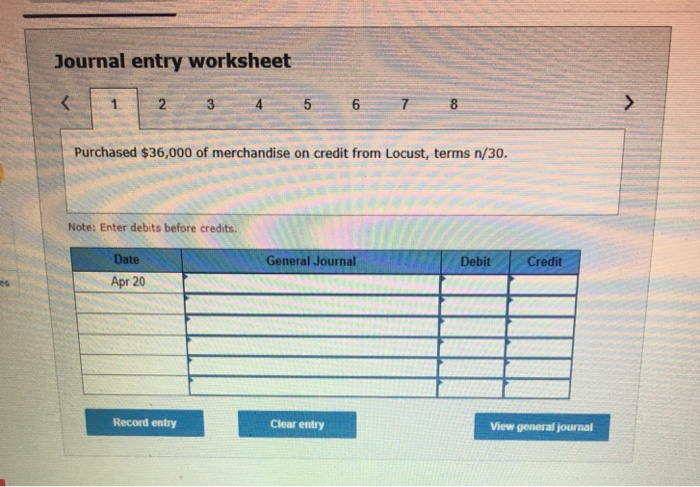# Required information [The following information applies to the questions displayed below) Tyrell Co. entered into the...

###### Question:Required information [The following information applies to the questions displayed below) Tyrell Co. entered into the following transactions involving short-term liabilities. Year 1 Apr. 20 Purchased $36,000 of merchandise on credit from Locust, terms n/30. May 19 Replaced the April 20 account payable to Locust with a 90-day, 9%,$35,000 note payable along with paying $1,880 in cash. July 8 Borrowed 557,000 cash from NBR Bank by signing a 120-day, 11%,$57,000 note payable. _?_ Paid the amount due on the note to Locust at the maturity date. Paid the amount due on the note to NBR Bank at the maturity date. Nov. 28 Borrowed $27,000 cash from Fargo Bank by signing a 60-day, 6%,$27,000 note payable. Dec. 31 Recorded an adjusting entry for accrued interest on the note to Fargo Bank. Year 2 _?_ Paid the amount due on the note to Fargo Bank at the maturity date. Required: 1. Determine the maturity date for each of the three notes described. Locust NBR Bank Fargo Bank Maturity date Prev 1 2 .5 of 6 Next >
Year 2 Paid the amount due on the note to Fargo Bank at the naturity date. 2. Determine the interest due at maturity for each of the three notes. (Do not round your intermediate cald year.) Principal X Rate x Time - Interest Locust NBR Bank Fargo Bank
3. Determine the interest expense recorded in the adjusting entry at the end of Year 1. (Do not round your Use 360 days a year.) Year End Accrual Required For: Principal * Rate Fargo Bank * Time % - Interest Interest to be accrued in Year 1 ces
Paid the amount due on the note to Fargo Bank at the naturity date. 4. Determine the interest expense recorded in Year 2. (Do not round intermediate calculations and ro nearest whole dollar. Use 360 days a year.) Year end accrual required for: Fargo Bank x Time Principal X Rate - Interest Interest to be recorded in Year 2
##### 1. Identify the most acidic proton in each molecule. Briefly explain your reasoning based on structure...
1. Identify the most acidic proton in each molecule. Briefly explain your reasoning based on structure (stating the pKa value is not enough, here. You must bring in factors related to ARIO). OH HACO NO2...
##### Please answer both with an explanation (14) For testing Ho : µ1 = µ2 versus H1...
please answer both with an explanation (14) For testing Ho : µ1 = µ2 versus H1 : µ1  µ2, a completely randomized design used two indpendent samples of sizes n = m = 10 and obtained t-statistics = -2.87. If the assumption of equal but unknown population variances is justif...
##### 1. What are the three classes of factors that influence perception? A) factors in the setting,...
1. What are the three classes of factors that influence perception? A) factors in the setting, factors in the environment, and factors in the motives B) factors in the perceiver, factors in the target, and factors in the situation C) factors in the character, factors in knowledge, and factors in exp...